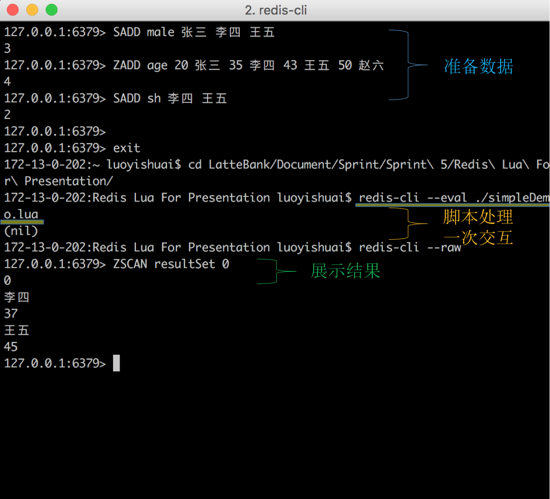# PHP 使用位运算实现四则运算的代码_php实例（1+2）+3-4*6/6 解：（先算括号中的（有冥方的先算冥方） 3+3-4*6/6 （再算乘除法，从左到右） 3+3-24/6 3+3-4 （最后是加减法，也是从左到右） 6-4 2⒈ 加法

<?php function add(\$summand, \$addend) { \$sum = \$summand ^ \$addend; // 判断进位 \$carry = \$summand & \$addend; while (\$carry <<= 1) { \$summand = \$sum; \$addend = \$carry; \$sum = \$summand ^ \$addend; \$carry = \$summand & \$addend; } return \$sum; }

⒉ 减法

<?php require 'addition.php'; function subtract(\$minuend, \$subtrahend) { // 先求得减数的补码，然后求和 \$subtrahend = add(~\$subtrahend, 1); return add(\$minuend, \$subtrahend); }

⒊ 乘法

0 0 1 1

×      1 0 1 0

――――――――――――

0 0 0 0

0 0 1 1 0

0 0 0 0 0 0

0 0 1 1 0 0 0

――――――――――――

0 0 1 1 1 1 0

<?php require 'addition.php'; function multiply(\$multiplicand, \$multiplicator) { // 判断符号位 \$flag = (\$multiplicand ^ \$multiplicator) < 0 ? false : true; // 被乘数和乘数取绝对值 \$multiplicand = \$multiplicand < 0 ? add(~\$multiplicand, 1) : \$multiplicand; \$multiplicator = \$multiplicator < 0 ? add(~\$multiplicator, 1) : \$multiplicator; \$product = 0; \$multiplicator = decbin(\$multiplicator); \$length = strlen(\$multiplicator); for (\$i = 0; \$i < \$length; \$i ++) { if (\$multiplicator[\$i]) { \$product += \$multiplicand << \$length - \$i - 1; } } if (! \$flag) { \$product = add(~\$product, 1); } return \$product; }

⒋ 除法

<?php require 'addition.php'; function divide(\$dividend, \$divisor) { // 判断符号位 \$flag = (\$dividend ^ \$divisor) < 0 ? false : true; // 取得被除数符号位 \$dividend_flag = \$dividend < 0 ? false : true; // 取绝对值 \$dividend = \$dividend < 0 ? add(~\$dividend, 1) : \$dividend; \$divisor = \$divisor < 0 ? add(~\$divisor, 1) : \$divisor; \$quotient = 0; \$remainder = 0; if (\$dividend < \$divisor) { // 被除数小于除数的情况 \$remainder = \$dividend; return 'quotient = ' . \$quotient . ' remainder = ' . \$remainder; } while (\$dividend >= \$divisor) { \$i = 0; \$mul_divisor = \$divisor; while (\$dividend >= (\$mul_divisor << 1)) { \$i ++; \$mul_divisor <<= 1; } \$dividend -= \$mul_divisor; \$quotient += 1 << \$i; } \$remainder = \$dividend; if (! \$flag) { \$quotient = add(~ \$quotient, 1); } if (! \$dividend_flag) { \$remainder = add(~\$remainder, 1); } return 'quotient = ' . \$quotient . ' remainder = ' . \$remainder; }

import java.text.DecimalFormat;import java.util.Scanner;public classZhidao {public static void main(String[]args){String condition=";Zhidao=newZhidao();do{Scanner scanner=new Scanner(System.in);try{System.out.print("请输入第一个数：");double x=scanner.nextDouble();System.out.print("请输入第二个数：");double y=scanner.nextDouble();System.out.print("请输入运算符：");String s=scanner.next();char z=s.charAt(0);.yunsuan(x,y,z);}catch(Exception e){System.out.println("请输入正确的数据！}System.out.print("是否继续？continue：继续，任意字符：结束");condition=scanner.next();}while("continue".equals(condition));}public static void yunsuan(double x,double y,Character z){DecimalFormat r=new DecimalFormat();r.applyPattern("#0.00");if(z.equals('+')){System.out.println(x+"+"+y+"="+r.format((x+y)));} else if(z.equals('-')){System.out.println(x+"-"+y+"="+r.format((x-y)));} else if(z.equals('*')){System.out.println(x+"*"+y+"="+r.format((x*y)));} else if(z.equals('/')){if(y=0){System.out.println("被除数不能为0");} else{System.out.println(x+"/"+y+"="+r.format((x/y)));}}else{System.out.println("无法识别改运算符");}}}内容来自www.zgxue.com请勿采集。

• 本文相关：
• 老生常谈php位运算的用途
• php巧妙利用位运算实现网站权限管理的方法
• php实现的简单四则运算计算器功能示例
• 用php实现的四则运算表达式计算实现代码
• php 实现收藏功能的示例代码
• php web环境和命令行环境下查找php.ini的位置
• php操作xml、读取数据和写入数据的实现代码
• php使html标签自动补全闭合函数代码
• mantis安装、配置和使用中的问题小结
• thinkphp调用sqlserver储存过程返回多个结果集
• laravel5.5 手动分页和自定义分页样式的简单实现
• php调用百度人脸识别接口查询数据库人脸信息实现验证登录功能
• php类中的魔术方法（magic method）简明总结
• ecshop 批量上传（加入自定义属性）
• 编写一个实现四则运算的JAVA程序
• PHP位运算符理解
• 按位运算作用是什么
• PHP位运算问题.
• 用位运算实现加倍操作，写出相应代码
• php一个表单里多个按钮,怎么知道是哪个对应的（简单的四则运算）
• 位运算符的问题，&不能进行int,float运算。为什么？
• 实现带有括号的四则运算。输入是一个带有括号的四则运算表达式，输出是计算得出的正确计算结果。
• 运算器的主要功能是（ ）
• c# 使用控制台程序编写一个简单的四则运算计算器
• 免责声明 - 关于我们 - 联系我们 - 广告联系 - 友情链接 - 帮助中心 - 频道导航